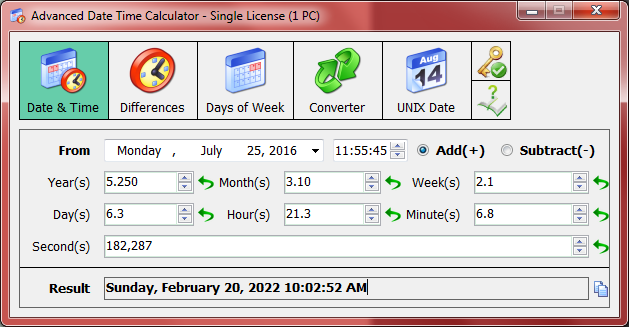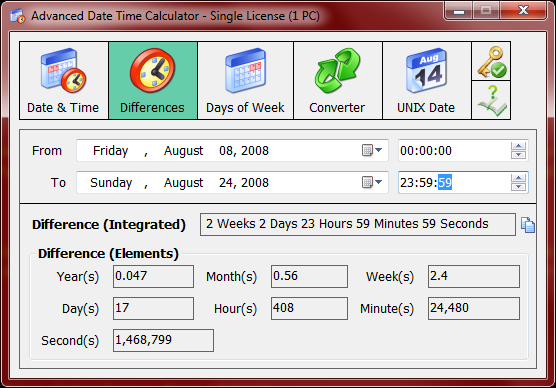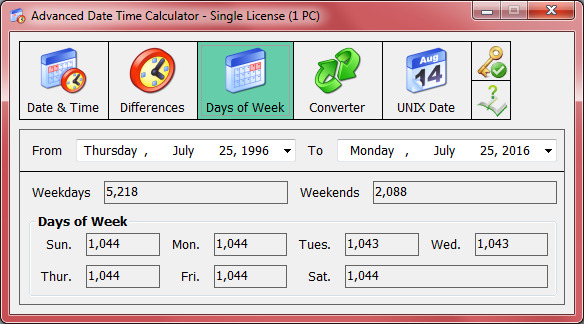1. Calculate the date by adding or subtracting years, months, weeks, days, hours, minutes or seconds. This calculator for time allows people to calculate what date it will be in a number of days after or before a special date. This date duration calculation is useful to calculating the timing involved in contracts or planning of various events.2. Calculate an event's duration like the number of days the Beijing Olympics 2008 lasted. This software can do date duration calculation that allows people to calculate what date it will be in a number of days after or before a special date, which is a useful date duration calculation to calculate the age of a person or how many days you have spent on certain things. And the time could also be converted into a year number.3. Calculate how many weekdays and weekends between two dates. If you want to know how many workdays between two dates or how many weekends you have in this term, you can try this calculator for time.This screenshot showed how many workdays between two dates.

4. Convert the complex units of time into a single unit (e.g. years or hours) by the same calculator for time.5. The UNIX timestamp conversion tool can convert UNIX days / seconds to / from a special normal date. Make time exchange a real cinch. Very professional for technical persons.If you're interest in it, please try this date and time calculator by the following button: